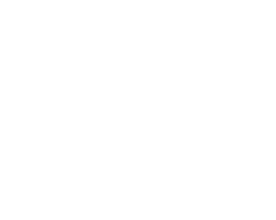October 19, 2023
October 19, 2023
October 19, 2023
###### Operating-Systems
October 19, 2023
 Question 12

The truth tablerepresents the Boolean function

 A X B X + Y C X ⊕ Y D Y
Question 12 Explanation:
f(X,Y) = XY’ + XY = X(Y’ + Y) = X
Question 12 Explanation:
f(X,Y) = XY’ + XY = X(Y’ + Y) = X